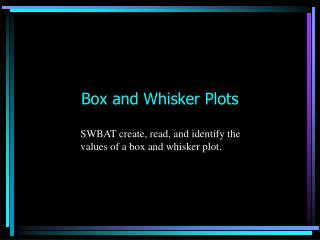# Box and Whisker Plots - PowerPoint PPT PresentationDownload PresentationBox and Whisker Plots

Box and Whisker PlotsDownload Presentation## Box and Whisker Plots

- - - - - - - - - - - - - - - - - - - - - - - - - - - E N D - - - - - - - - - - - - - - - - - - - - - - - - - - -
##### Presentation Transcript

1. Box and Whisker Plots SWBAT create, read, and identify the values of a box and whisker plot.

2. Finish Data Collection

3. A box plot summarizes data using the median, upper and lowerquartiles, and the extreme (least and greatest) values. It allows you to see important characteristics of the data at a glance.

4. Example Problem: The gas mileages in miles per gallon (mpg) of 4-cylinder manual transmission cars are in a table on the next slide

5. MPG of 4-cylinder cars

6. 24 25 28 28 29 29 29 30 30 31 31 32 32 32 33 34 37 38 38 39 42 44 44 44 To make a box plot, organize the data by arranging the data in order from least to greatest.

7. 24 25 28 28 29 29 29 30 30 31 31 32 32 32 33 34 37 38 38 39 42 44 44 44Find the median of the data. It is 32This divides the data in half. The lower half : 24 25 28 28 29 29 29 30 30 31 31 32 and the upper half: 32 32 33 34 37 38 38 39 42 44 44 44

8. Find the median of the top half of the data. 32 32 33 34 37 38 38 39 42 44 44 44This is called the high median, upper quartile or quartile 3. It is 38.

9. Take the lower half of the data and find the median of it.24 25 28 28 29 29 29 30 30 31 31 32This data, 29, is called the low median, lower quartile or quartile 1.

10. Next, find the lower and upper extremes. This simply means the lowest data, 24, and the highest data, 44.Let’s organize all 5 pieces of data together so we can see them.

11. Lower extreme = 24Lower quartile(Q1) =29Median (Q2) = 32Upper quartile(Q3) =38Upper extreme(Q4)=44The data is now divided into quartiles(4ths) so each quartile represents one-fourth of the data.

12. Next, make a number line that will best display the 5 pieces of data (24 29 32 38 44)

13. Place a dot above the number line to show the lower extreme and one for the upper extreme.

14. Put a vertical slash above the number line for the median and one for the lower and upper quartiles.

15. Enclose the vertical slashes into a box. Draw a line from the right center of the box to the upper extreme and one from the lower end of the box to the lower extreme, forming the whiskers.

16. You must label the number line to tell what the data represents.Miles per gallon (mpg)

17. All graphs must have a title that clearly represents what your graph is showing. Miles per Gallon of 4-cylinder Cars Miles per gallon (mpg)

18. Interpreting the Box Plot: Study your Box and Whisker Plot to determine what it is telling you. Make a statement about what it is saying, then support the statement with facts from your graph.

19. You should include the following in your interpretation: • Range or spread of the data and what it means to your graph • Quartiles—compare them. What are they telling you about the data? • Median- this is an important part of the graph, and should be an important part of the interpretation. • Percentages should be used to interpret the data, where relevant.

20. We will now interpret the data we have on mpg of 4-cylinder cars. We will do this step-by-step, then put all the interpretation together as our final summary.

21. Miles per Gallon of 4-cylinder Cars Miles per gallon (mpg)

22. Make a statement about what it is saying, then support the statement with facts from your graph: The Box and Whisker Plot clearly shows that are a lot of different gas mileages on various 4-cylinder vehicles

23. Miles per Gallon of 4-cylinder Cars Miles per gallon (mpg)

24. Range or spread of the data and what it means to your graph The mileage ranged from 24 miles per gallon(mpg) to a high of 44 mpg. This is a 20 miles per gallon spread, which in car mileage is quite a bit of difference.

25. Quartiles—compare them. What are they telling you about the data? The first quartile reads as 32 mpg which means that 75% of the vehicles in this study got 32 mpg or more. The 3rd quartile tells us that 25% of these cars got 38 mpg or higher which is really good mileage.

26. Median- this is an important part of the graph, and should be an important part of the interpretation. The median cuts the data in half. The median is 32 mpg. Therefore half the cars in the study received 32 mpg or higher.